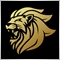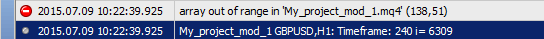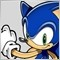Need little help with Array out of range - page 2Moderator
12468

So if you put in a Print command to reveal the value of i

for (i = iBars(NULL, TimeFrame) - 1 - prev_calculated; i > 0; i--) {
if(TimeFrame == Period()) {
Up  = iHigh(NULL, TimeFrame, iHighest(NULL, TimeFrame, MODE_HIGH, Range, i));
Dn  = iLow (NULL, TimeFrame, iLowest (NULL, TimeFrame, MODE_LOW,  Range, i));

} else if(TimeFrame > Period()) {

Print("Timeframe: ",TimeFrame," i= ",i);

hightfshift = iBarShift(NULL, TimeFrame, Time[i], true);
if (hightfshift == -1) continue;
Up  = iHigh(NULL, TimeFrame, iHighest(NULL, TimeFrame, MODE_HIGH, Range, hightfshift));
Dn  = iLow (NULL, TimeFrame, iLowest (NULL, TimeFrame, MODE_LOW,  Range, hightfshift));
} else if(TimeFrame < Period()) {

Print("Timeframe: ",TimeFrame," i= ",i);

smalltfshift = iBarShift(NULL, TimeFrame, Time[i], true);
if (smalltfshift == -1) continue;
timeshift  = iTime(NULL, TimeFrame, smalltfshift);
xshift = iBarShift(NULL, TimeFrame, timeshift, true);

//if(xshift<0){xshift=0;}
//if(smalltfshift<0){smalltfshift=0;}
//if(timeshift<0){timeshift=0;}

Up  = iHigh(NULL, TimeFrame, iHighest(NULL, TimeFrame, MODE_HIGH, Range, xshift));
Dn  = iLow (NULL, TimeFrame, iLowest (NULL, TimeFrame, MODE_LOW,  Range, xshift));
}

Resistance[i] = Up;
Support[i]= Dn;

}

it shows you the value of i363

ok, I need to go now, I will try to understand the source and I will tell you later

Thanks for you help MarcoModerator
12468

See if you read the pink Time Array

Time[]

it will read the time of the current chart, and not of your Timeframe.

if you want to read it of the Timeframe period use

iTime(....
for (i = iBars(NULL, TimeFrame) - 1- prev_calculated; i > 0; i--) {
if(TimeFrame == Period()) {
Up  = iHigh(NULL, TimeFrame, iHighest(NULL, TimeFrame, MODE_HIGH, Range, i));
Dn  = iLow (NULL, TimeFrame, iLowest (NULL, TimeFrame, MODE_LOW,  Range, i));

} else if(TimeFrame > Period()) {

Print(" prev_calculated: ",prev_calculated,"Timeframe: ",TimeFrame," i= ",i," Bars on chart: ",iBars(NULL, TimeFrame));

hightfshift = iBarShift(NULL, TimeFrame, iTime(NULL,TimeFrame,i));
if (hightfshift == -1) continue;
Up  = iHigh(NULL, TimeFrame, iHighest(NULL, TimeFrame, MODE_HIGH, Range, hightfshift));
Dn  = iLow (NULL, TimeFrame, iLowest (NULL, TimeFrame, MODE_LOW,  Range, hightfshift));
} else if(TimeFrame < Period()) {

Print(" prev_calculated: ",prev_calculated,"Timeframe: ",TimeFrame," i= ",i," Bars on chart: ",iBars(NULL, TimeFrame));

smalltfshift = iBarShift(NULL, TimeFrame, iTime(NULL,TimeFrame,i));
if (smalltfshift == -1) continue;
timeshift  = iTime(NULL, TimeFrame, smalltfshift);
xshift = iBarShift(NULL, TimeFrame, timeshift, true);

//if(xshift<0){xshift=0;}
//if(smalltfshift<0){smalltfshift=0;}
//if(timeshift<0){timeshift=0;}

Up  = iHigh(NULL, TimeFrame, iHighest(NULL, TimeFrame, MODE_HIGH, Range, xshift));
Dn  = iLow (NULL, TimeFrame, iLowest (NULL, TimeFrame, MODE_LOW,  Range, xshift));
}

Resistance[i] = Up;
Support[i]= Dn;

}39010

Marco vd Heijden:

See if you read the pink Time Array

it will read the time of the current chart, and not of your Timeframe.

if you want to read it of the Timeframe period use

Hi Marco, can I suggest you to highlight the changes you made when you post code (I edited your post as an example). Thank you.Moderator
12468

Alain Verleyen:
Hi Marco, can I suggest you to highlight the changes you made when you post code (I edited your post as an example). Thank you.
ah ok thanks i have no idea how to do that but i will find it thanks.39010

Marco vd Heijden:
ah ok thanks i have no idea how to do that but i will find it thanks.

Ah sorry. I used the "background color" button :Moderator
12468

Alain Verleyen:

Ah sorry. I used the "background color" button :

test

test
oh wow thanks :) im learning something new every day !!363

Hi Marco, finally I can fixed it

Thanks again Marco (Y)

for (i = iBars(NULL, TimeFrame) - 1 - prev_calculated; i > 0; i--) {
if(TimeFrame == Period()) {
Up  = iHigh(NULL, TimeFrame, iHighest(NULL, TimeFrame, MODE_HIGH, Range, i));
Dn  = iLow (NULL, TimeFrame, iLowest (NULL, TimeFrame, MODE_LOW,  Range, i));
} else if(TimeFrame > Period()) {
hightfshift = iBarShift(NULL, TimeFrame, Time[i], true);
if (hightfshift == -1) continue;
Up  = iHigh(NULL, TimeFrame, iHighest(NULL, TimeFrame, MODE_HIGH, Range, hightfshift));
Dn  = iLow (NULL, TimeFrame, iLowest (NULL, TimeFrame, MODE_LOW,  Range, hightfshift));
} else if(TimeFrame < Period()) {
if (iTime(NULL,0,i) != iTime(NULL,TimeFrame,i)) timex = iTime(NULL,0,i);
smalltfshift = iBarShift(NULL, TimeFrame, timex, true);
if (smalltfshift == -1) continue;
timeshift  = iTime(NULL, TimeFrame, smalltfshift);
xshift = iBarShift(NULL, TimeFrame, timeshift, true);
Up  = iHigh(NULL, TimeFrame, iHighest(NULL, TimeFrame, MODE_HIGH, Range, xshift));
Dn  = iLow (NULL, TimeFrame, iLowest (NULL, TimeFrame, MODE_LOW,  Range, xshift));
}

Resistance[i] = Up;
Support[i]= Dn;

}